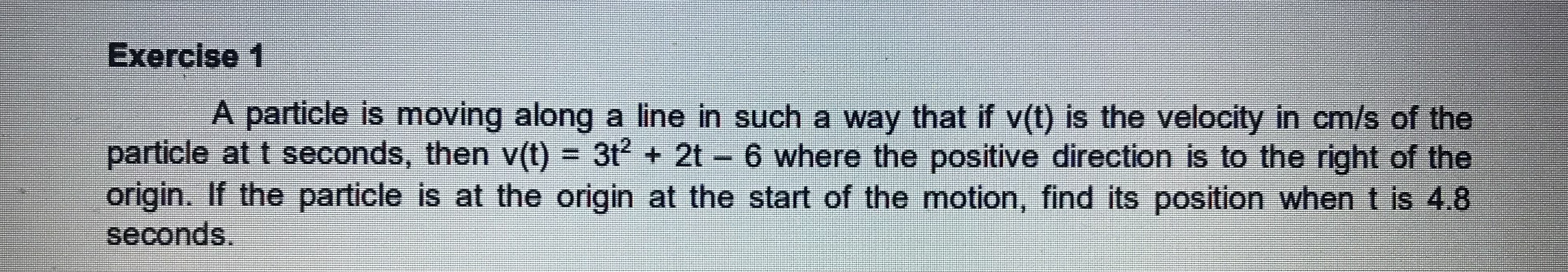### ¿Todavía tienes preguntas de matemáticas?

Pregunte a nuestros tutores expertos
Algebra
PreguntaA particle is moving along a line in such a way that if $$v ( t )$$ is the velocity in $$cm / s$$ of the particle at $$t$$ seconds, then $$v ( t ) = 3 t ^ { 2 } + 2 t - 6$$ where the positive direction is to the right of the origin. If the particle is at the origin at the start of the motion, find its position when $$t$$ is $$4.8$$ seconds.

$$3 \cdot 4.8 ^ { 2 } + 2 \cdot 4.8 - 6 = 72.72$$

Steps

$$3 \cdot 4.8 ^ { 2 } + 2 \cdot 4.8 - 6$$

Multiply the numbers: $$2 \cdot 4.8 = 9.6$$

$$= 3 \cdot 4.8 ^ { 2 } + 9.6 - 6$$

Add $$/$$ Subtract the numbers: $$9.6 - 6 = 3.6$$

$$= 3 \cdot 4.8 ^ { 2 } + 3.6$$

$$3 \cdot 4.8 ^ { 2 } = 69.12$$

$$= 69.12 + 3.6$$

Add the numbers: $$69.12 + 3.6 = 72.72$$

$$= 72.72$$

Solución
View full explanation on CameraMath App.